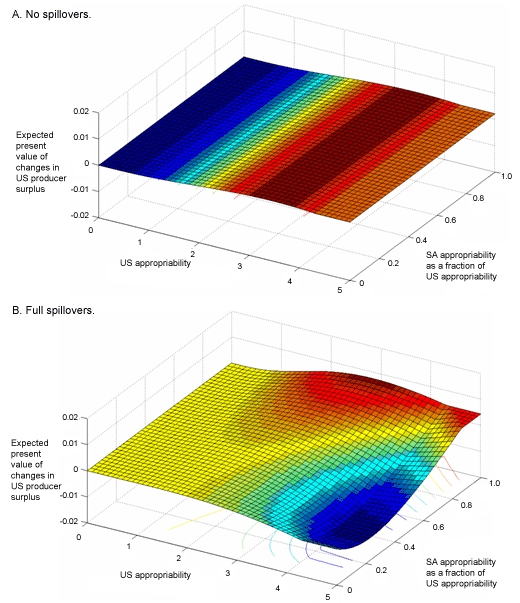# Why We Use Percentage Change Rather Than Absolute Change in Elasticity

162

## WHY WE USE PERCENTAGE CHANGE RATHER THAN ABSOLUTE CHANGE IN ELASTICITY?

1. By using percentage changes and proportions we can avoid the problem of comparison in two different quantitative variables i-e Qd is measured in units and Price is measured in rupees. So by calculating percentages we can avoid the problem of unit conversion into rupees.

2. It helps us avoid that of what size of units to be changed i-e A jump from Rs.2 to Rs.4 could be described as a 100% increase or as an increase of Rs.2. but by using percentages we can avoid this problem because both gives the same answer.

3. It also helps how to define big or small changes. By looking at Rs.2 or Rs.4, we can’t say that it is a big change or a small change. But if we translate it in the form of percentages then it becomes 100% which is a big change. Virtual University